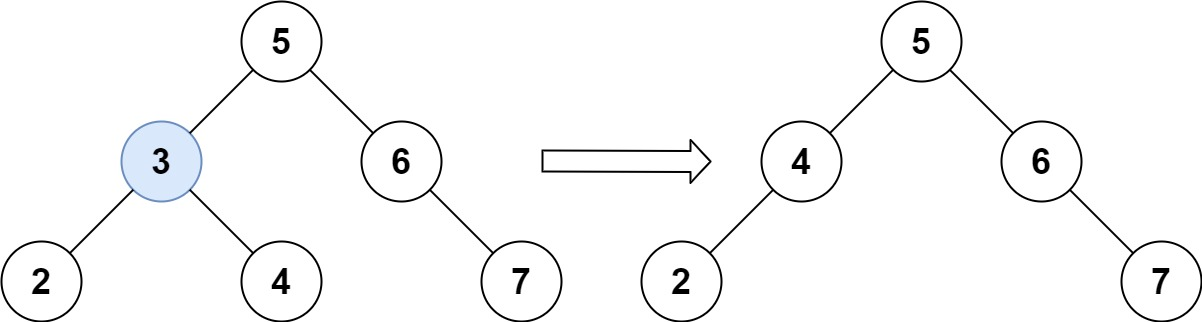# 450. Delete Node in a BST

Medium
Given a root node reference of a BST and a key, delete the node with the given key in the BST. Return the root node reference (possibly updated) of the BST.
Basically, the deletion can be divided into two stages:
1. 1.
Search for a node to remove.
2. 2.
If the node is found, delete the node.
Example 1:Input: root = [5,3,6,2,4,null,7], key = 3
Output:
[5,4,6,2,null,null,7]
Explanation:
Given key to delete is 3. So we find the node with value 3 and delete it.
One valid answer is [5,4,6,2,null,null,7], shown in the above BST.
Please notice that another valid answer is [5,2,6,null,4,null,7] and it's also accepted.
Example 2:
Input: root = [5,3,6,2,4,null,7], key = 0
Output:
[5,3,6,2,4,null,7]
Explanation:
The tree does not contain a node with value = 0.
Example 3:
Input: root = [], key = 0
Output:
[]
Constraints:
• The number of nodes in the tree is in the range `[0, 104]`.
• `-105 <= Node.val <= 105`
• Each node has a unique value.
• `root` is a valid binary search tree.
• `-105 <= key <= 105`
Follow up: Could you solve it with time complexity `O(height of tree)`?

### 解題

/**
* Definition for a binary tree node.
* type TreeNode struct {
* Val int
* Left *TreeNode
* Right *TreeNode
* }
*/
func deleteNode(root *TreeNode, key int) *TreeNode {
if root == nil {
return root
}
if key < root.Val {
root.Left = deleteNode(root.Left, key)
} else if key > root.Val {
root.Right = deleteNode(root.Right, key)
} else {
if root.Left == nil && root.Right == nil { //為left node ，直接回傳nil即可
return nil
}
if root.Left == nil { // 左子樹為空，回傳右子樹
temp := root.Right
root.Right = nil
return temp
}
if root.Right == nil { //右子樹為空，回傳左子樹
temp := root.Left
root.Left = nil
return temp
}
temp := succesor(root.Right) //從右邊選出最小的值作為繼任的節點
root.Val = temp.Val
root.Right = deleteNode(root.Right, temp.Val) //將繼任的節點從右邊刪除
}
return root
}
func succesor(root *TreeNode) *TreeNode {
for root.Left != nil {
root = root.Left
}
return root
}